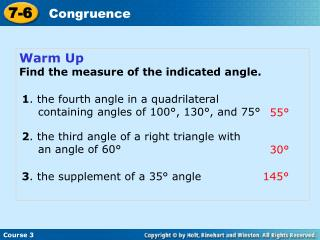DownloadDownload PresentationWarm Up Find the measure of the indicated angle.

# Warm Up Find the measure of the indicated angle.

Download Presentation## Warm Up Find the measure of the indicated angle.

- - - - - - - - - - - - - - - - - - - - - - - - - - - E N D - - - - - - - - - - - - - - - - - - - - - - - - - - -
##### Presentation Transcript

1. 7-6 Congruence Course 3 Warm Up Find the measure of the indicated angle. 1. the fourth angle in a quadrilateral containing angles of 100°, 130°, and 75° 55° 2. the third angle of a right triangle with an angle of 60° 30° 3. the supplement of a 35° angle 145°

2. 7-6 Congruence Course 3 Problem of the Day The measure of ABC is 14° less than the measure of its complement, CBD. What is the measure of each angle? mABC = 38°; mCBD = 52°

3. 7-6 Congruence Course 3 TB P. 354-357 Learn to use properties of congruent figures to solve problems.

4. 7-6 Congruence Course 3 Vocabulary correspondence

5. 7-6 Congruence Course 3 A correspondence is a way of matching up two sets of objects. If two polygons are congruent, all of their corresponding sides and angles are congruent. In a congruence statement, the vertices in the second polygon are written in order of correspondence with the first polygon.

6. 7-6 Congruence Helpful Hint Marks on the sides of a figure can be used to show congruence. AB@QR (1 mark) BC@PR (2 mark) AC@PQ (3 mark) __ __ __ __ __ __ Course 3

7. 7-6 Congruence 55 55 Course 3 Additional Example 1A: Writing Congruent Statements Write a congruence statement for each pair of polygons. The first triangle can be named triangle ABC. To complete the congruence statement, the vertices in the second triangle have to be written in order of the correspondence. A@Q, so A corresponds to Q. B@R, so B corresponds to R. C@P, so C corresponds to P. The congruence statement is triangle ABC@ triangle QRP.

8. 7-6 Congruence Course 3 Additional Example 1B: Writing Congruent Statements Write a congruence statement for each pair of polygons. The vertices in the first pentagon are written in order around the pentagon starting at any vertex. D@M, so D corresponds to M. E@N, so E corresponds to N. F@O, so F corresponds to O. G@P, so G corresponds to P. H@Q, so H corresponds to Q. The congruence statement is pentagon DEFGH@ pentagon MNOPQ.

9. 7-6 Congruence WX @ KL a + 8 = 24 –8 –8 a = 16 Course 3 Additional Example 2A: Using Congruence Relationships to Find Unknown Values In the figure, quadrilateral VWXY@ quadrilateral JKLM. Find a. Subtract 8 from both sides.

10. 7-6 Congruence ML @ YX 6b = 30 6b = 30 6 6 Course 3 Additional Example 2B: Using Congruence Relationships to Find Unknown Values In the figure, quadrilateral VWXY@ quadrilateral JKLM. Find b. Divide both sides by 6. b = 5

11. 7-6 Congruence J @V 5c = 85 5c = 85 5 5 Course 3 Additional Example 2C: Using Congruence Relationships to Find Unknown Values In the figure, quadrilateral VWXY@ quadrilateral JKLM. Find c. Divide both sides by 5. c = 17

12. 7-6 Congruence 1. Find XY. 3. Find CD. Course 3 Lesson Quiz In the figure, WXYZ@ABCD 10 80° 2. Find mB. 8 90° 4. Find mZ.